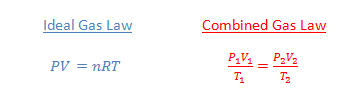## Ideal Gas Law vs. Combined Gas Law

When you are doing gas law problems, your first decision should be whether to use the Ideal gas law or the combined gas law. How do you know which to use?The Ideal Gas Law is used when you have one set of conditions for your system. Then if you know any three of the values for pressure, volume, moles and temperature, you can solve for the unknown quantity. The Combined Gas Law is used when you have two sets of conditions. In other words, you know the initial pressure, volume and temperature, and two of those quantities change to a new set of conditions. Then you can calculate the new value of the third quantity.

Example 1: What is the volume of a container that holds 0.561 mol of a gas at 1.15 atm at 27°C?

Answer: This problem describes a single set of conditions, so use the Ideal Gas Law:$PV=nRT$$(1.15 \, atm)(V)=(0.561 \, mol) \! \left ( 0.0821 \, \frac{\ell \cdot atm}{mol \cdot K} \right ) \! (300 \, K)$$V=12.0 \, L$

Example 2: A 3.00 L container holds an ideal gas at STP. What will the new pressure be if the volume is increased to 3.75 L and the temperature is increased to 500 K?

Answer: This problem describes an initial condition and a new set of conditions, so use the combined gas law:$\dfrac{P_1V_1}{T_1}= \dfrac{P_2V_2}{T_2}$$\dfrac{(1.00 \, atm)(3.00 \, L)}{273 \, K}= \dfrac{(P)(3.75 \, L)}{500 \, K}$$P=1.47 \, atm$

Chemistry
Blue Taste Theme created by Jabox